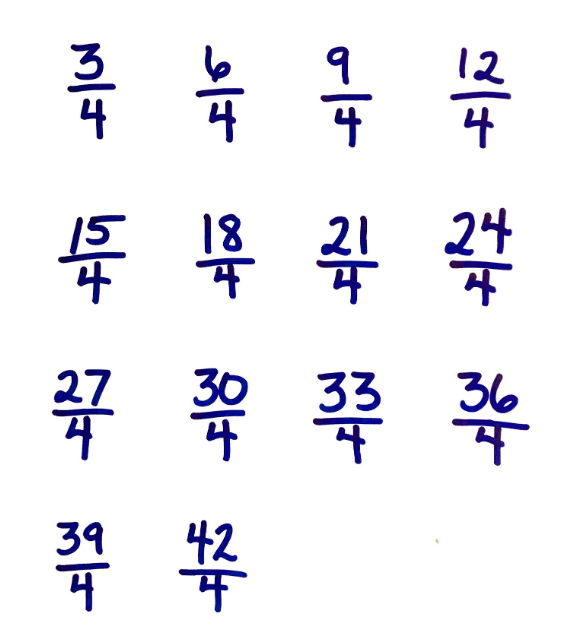By William McCallumIn one of our professional development workshops, there is an activity in which the facilitator asks teachers to skip count by $\frac34$. The facilitator records the count, $\frac34$, $\frac64$, $\frac94$, . . . and then asks for patterns they notice in the recording. In a recent workshop, a group of grade 5 teachers noticed that the numerator increased by 3 each time but that the denominator remained unchanged. When the facilitator asked why, they could easily explain the number of pieces were increasing in the numerator, but couldn’t really give an explanation for the denominator other than “it is just always out of 4.” The funny thing is, they weren’t saying “3 out of 4, 6 out of 4, 9 out of 4” when they skip counted. They were saying “3 fourths, 6 fourths, 9 fourths” and writing it in fraction notation. The key to understanding why the denominator stayed the same was hidden in plain sight, in the very language they were using to name the fractions.

To understand this, recall that in the standards fractions are built up out of unit fractions. In grades 1–2 students learn that numbers can be built up out of ones, tens, hundreds, etc. They learn to see 43 as 4 tens and 3 ones. They see ten as a single unit made by bundling together 10 ones, and they learn to unbundle that unit when necessary for addition or subtraction.

In grade 3, students start to see the number one itself as a bundle of smaller units. Their rulers become subdivided into halves or fourths of an inch. On their number line diagrams they see the length from 0 to 1 subdivided into smaller equal lengths, say thirds or fifths. They learn to  see $\frac13$ not as “one out of three” but as a piece of a certain size, three of which make a whole. And then they build other fractions out of pieces; $\frac53$ is literally five thirds, the number you get put putting 5 pieces called $\frac13$ together on the number line.

The work in geometry in grades 1–2 has prepared them for this thinking. To quote from the latest version of the fractions progression:

“. . . first graders might put two congruent isosceles triangles together with the explicit purpose of making a rhombus. In this way, they learn to perceive a composite shape as a unit—a single new shape, e.g., recognizing that two isosceles triangles can be combined to make a rhombus, and simultaneously seeing the rhombus and the two triangles. . . . [they] use fraction language to describe partitions of simple shapes into equal shares—halves, fourths, and quarters in grade 1, extending into thirds in grade 2.”

Where does the “out of” language come from? It is fairly natural language to use when you are describing fractions less than 1. You divide a rectangle into 4 equal parts and shade 3 out of the 4 to represent the fraction $\frac34$. It is also natural when using fractions to describe sets: 3 out of the 4 roses were red, so $\frac34$ of the roses were red. The danger here is that students might not see beyond the formal process: count the red roses, count all the roses, then put the two numbers together. It is not a coincidence that the standards do not use set representations to introduce fractions, but rather use measurement representations (length or area) where the whole is more easily seen as one thing.

So perhaps one way to understand fractions better is to simply listen to ourselves when we name them. When we say “five sixteenths” we are not only naming a particular fraction, we are giving a constructive definition for it: you can figure out how big $\frac{5}{16}$ is by taking a unit called a sixteenth, 16 of which make a whole, and putting 5 of them together. It doesn’t always happen that our names for things describe their mathematical meaning, but this is one case where it does.

Here’s the record of the skip counting from the beginning of this post.What patterns do you notice? How would you explain why these patterns occur? Do the names for these numbers help reveal the mathematical underpinnings?

### Next Step

Can you think of an example where the words we use for a number get in the way of its mathematical meaning?